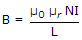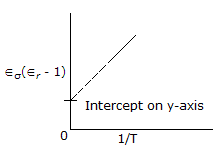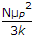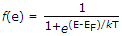# Electronics and Communication Engineering - Materials and Components

### Exercise :: Materials and Components - Section 1

41.

If n denotes principal quantum number, the angular quantum number l = 0, 1... (n - 1).

 A. True B. False

Explanation:

Angular quantum number determines the angular momentum of electron.

42.

Assertion (A): In a perfect capacitor, the current density is given by ω ∈0E0'rcos(ωt + 90°), where ∈r' is real part of dielectric constant.

Reason (R): In a perfect capacitor, dielectric losses are zero.

 A. Both A and R are true and R is correct explanation of A B. Both A and R are true but R is not correct explanation of A C. A is true but R is false D. A is false but R is true

Explanation:

If dielectric losses are zero, the current in capacitor leads the applied voltage by 90°.

Also imaginary part of is ∈r is zero.

43.

Assertion (A): For a solenoid having N turns,.

Reason (R): For all magnetic materials, B increases linearly with I.

 A. Both A and R are true and R is correct explanation of A B. Both A and R are true but R is not correct explanation of A C. A is true but R is false D. A is false but R is true

Explanation:

Increase of B with I is not linear. Hence R is false.

44.

Assertion (A): From the slope of the line in Figure, we can find permanent dipole moment of the molecules.

Reason (R): Total polarization of a polyatomic gas is given by P = N(ae + ai + μ2p / 3kT)E.A. Both A and R are true and R is correct explanation of A B. Both A and R are true but R is not correct explanation of A C. A is true but R is false D. A is false but R is true

Explanation:

The slope of the line in figure is.

Since the total polarization is as given by the expression, we can find the permanent dipole moment of the molecules from the slope.

45.

Assertion (A): If E is available energy state eV and EF is Fermi level, then probability of energy state E being occupied = 0.5.

Reason (R):.

 A. Both A and R are true and R is correct explanation of A B. Both A and R are true but R is not correct explanation of A C. A is true but R is false D. A is false but R is true